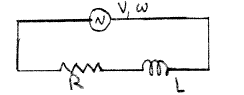# Problem: A series ac circuit has a source with voltage amplitude V = 120 V and angular frequency ω = 50 rad/s, a resistor with R = 15 Ω and an inductor with  L = 0.40 H. What is the current amplitude?

###### FREE Expert Solution
90% (471 ratings)
###### Problem Details

A series ac circuit has a source with voltage amplitude V = 120 V and angular frequency ω = 50 rad/s, a resistor with R = 15 Ω and an inductor with  L = 0.40 H. What is the current amplitude?Frequently Asked Questions

What scientific concept do you need to know in order to solve this problem?

Our tutors have indicated that to solve this problem you will need to apply the Impedance in AC Circuits concept. You can view video lessons to learn Impedance in AC Circuits. Or if you need more Impedance in AC Circuits practice, you can also practice Impedance in AC Circuits practice problems.

How long does this problem take to solve?

Our expert Physics tutor, Juan took 2 minutes and 18 seconds to solve this problem. You can follow their steps in the video explanation above.

What professor is this problem relevant for?

Based on our data, we think this problem is relevant for Professor Lyuksyutov's class at TAMU.